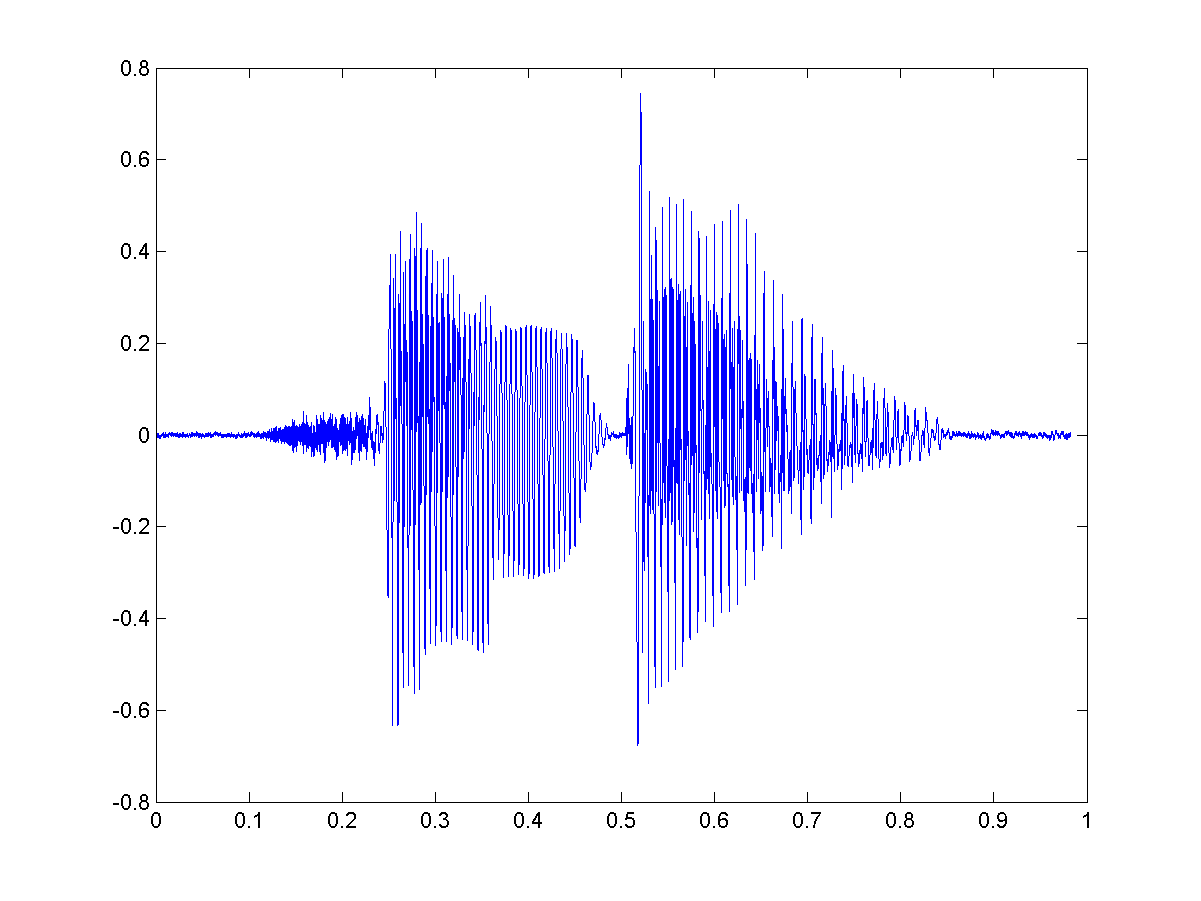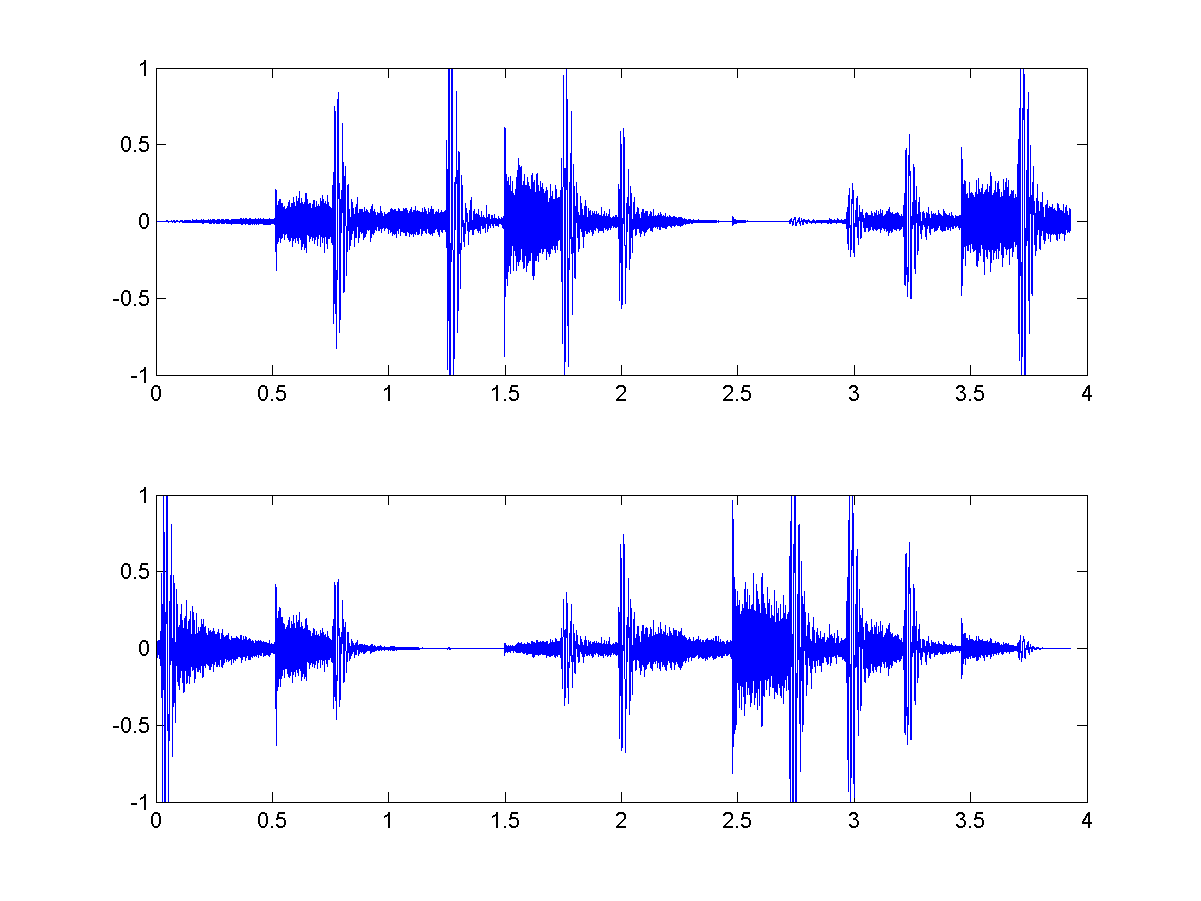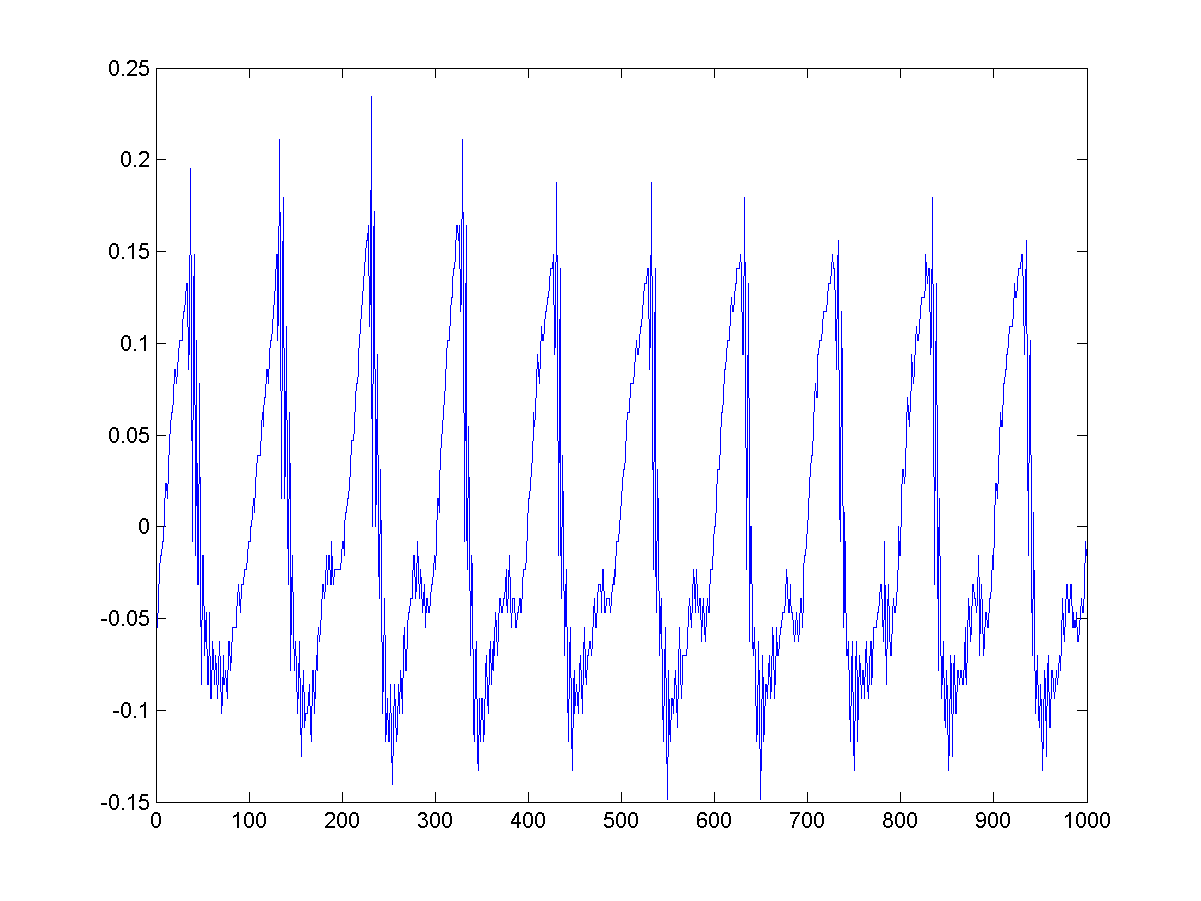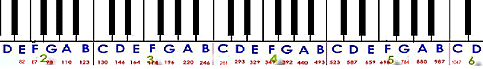On the Windows platform, the most common file extension for audio files is "wav". MATLAB can read such wave files via the command "wavread". The following example reads the wave file "sunday.wav" and display its waveform directly.

Example 1: matlab4asp/readWave01.m[y, fs]=wavread('sunday.wav'); sound(y, fs); % Playback of the sound data (播放此音訊) time=(1:length(y))/fs; % Time vector on x-axis (時間軸的向量) plot(time, y); % Plot the waveform w.r.t. time (畫出時間軸上的波形)In the above example, "fs" is the sample rate which is 16000 in this case. This indicates that there are 16000 samples per second when the clip was recorded. The vector "y" is a column vector containing the samples of the speech signals. We can use "sound(y, fs)" to play the audio signals read from the file. "time" is a time vector in which each element corresponds to the time of each sample. Therefore we can plot "y" against "t" to show the waveform directly.

Most audio signals are digitized to have a bit resolution of 8 or 16 bits. If we want to know the bit resolution of the input wave file, we can use an extra output arguments to "wavread" to obtain the information, such as

Moreover, if we want to know the time duration of a stream of audio signals, we can use "length(y)/fs" directly. The following example can obtain most of the important information of the wave file "welcome.wav".

Example 2: matlab4asp/readWave02.mfileName='welcome.wav'; [y, fs, nbits]=wavread(fileName); fprintf('Information of the sound file "%s":\n', fileName); fprintf('Duration = %g seconds\n', length(y)/fs); fprintf('Sampling rate = %g samples/second\n', fs); fprintf('Bit resolution = %g bits/sample\n', nbits);Information of the sound file "welcome.wav": Duration = 1.45134 seconds Sampling rate = 11025 samples/second Bit resolution = 8 bits/sample

From the above example, it can be observed that all the audio signals are between -1 and 1. However, each sample point is represented by an 8-bit interger. How are they related? First of all, we need to know the following convention:
1. If a wave file has a bit resolution of 8 bits, then each sample point is stored as an unsigned integer between 0 and 255 (= 2^8-1).
2. If a wave file has a bit resolution of 16 bits, then each sample point is stored as an unsigned integer between -32768 (= 2^16/2) and 32767 (= 2^16/2-1).
Since almost all variables in MATLAB have the data type of "double", therefore all samples are converted into a floating-point number between -1 and 1 for easy manipulation. Therefore to retrieve the original integer values of the audio signals, we can proceed as follows.
1. For 8-bit resolution, we can multiply "y" (the value obtained by wavread) by 128 and then plus 128.
2. For 16-bit resolution, we can multiply "y" (the value obtained by wavread) by 32768.
Here is an example.

Example 3: matlab4asp/readWave03.mfileName='welcome.wav'; [y, fs, nbits]=wavread(fileName); y0=y*(2^nbits/2)+(2^nbits/2); % y0 is the original values stored in the wav file (y0 是原先儲存在音訊檔案中的值) difference=sum(abs(y0-round(y0))) difference = 0

In the above example, the difference is zero, indicating the retrived y0 contains no fractional parts. Moreover, to increase code generality, we use 2^nbits/2 instead of 128.

Hint
In fact, it is possible to obtain the samples in native data formats directly. Please try "doc wavread" within MATLAB to view the details.

We can also use the command "wavread" to read a stereo wave file. The returned variable will be a matrix of 2 columns, each containing the audio signals from a single channel. Example follows.

Example 4: matlab4asp/readWave04.mfileName='flanger.wav'; [y, fs]=wavread(fileName); % Read wave file (讀取音訊檔) sound(y, fs); % Playback (播放音訊) left=y(:,1); % Left channel (左聲道音訊) right=y(:,2); % Right channel (右聲道音訊) subplot(2,1,1), plot((1:length(left))/fs, left); subplot(2,1,2), plot((1:length(right))/fs, right);In the above example, MATLAB will read the wave file "flanger.wav", play the stereo sound, and plot two streams of audio signals in two subplots. Since the intensities of these two channels are more or less complemntary to each other, which gives an illusion that the sound source is moving back and forth between two speakers. (A quiz: how do you create such effect given a single stream of audio signals?)

If the wave file is too large to be read into memory directly, we can also use "wavread" to read a part of the audio signals directly. See the following example.The waveform in the above example represent the vowel part of the second Chinese character "迎" in the original utterance of "歡迎光臨" (welcome). It is obvious that the waveform contain a fundamental period of about 100 samples, corresponding to a time duration of 100/fs = 0.0091 seconds = 9.1 ms. This corresponds to a pitch frequency of 11025/100 = 110.25 Hz. This pitch is very close to two octave down the central la, or the 5th white key counting from the left.The perception of pitch of human ear is proportional to the logrithm of the fundamental frequency. The central la of a piano has a fundamental frequency of 440 Hz. One octave above it is 880 Hz, while one octave below it is 220 Hz. Each octave in the piano keyboard contains 12 keys, including 7 white keys and 5 black ones, corresponding to 12 semitones within a octave. If we adopt the standard of MIDI files, the semitone of the central la is 69 with a fundamental frequency of 440. Therefore we can have a formula to convert a frequency into a semitone:

semitone = 69 + 12*log2(freq/440)
The process of computing the pitch contour of audio signals is usually called "pitch tracking". Pitch tracking is an important operation for applications such as text-to-speech synthesis, tone recognition and melody recognition. We shall introduce more methods for pitch tracking in the following chapters.

If we want to obtain more information about a wave file, we can retrieve it from the 4th output arguments of the command "wavread", as follows.

Example 6: matlab4asp/readWave06.m[y, fs, nbits, opts]=wavread('flanger.wav'); opts.fmt ans = wFormatTag: 1 nChannels: 2 nSamplesPerSec: 22050 nAvgBytesPerSec: 88200 nBlockAlign: 4 nBitsPerSample: 16

In the above example, some quantities are explained next.
1. wFormatTag is the format tag of the wave file.
2. nChannels is the number of channels.
3. nSamplePerSec is the number of samples per second, which is equal to the samping rate 22050.
4. nAveBytesPerSec is the number of bytes per second. In this case, since we have two channels and the bit resolution is 2 bytes, therefore we have 22050*4 = 88200.
5. nBlockAlign is equal to the rato between nAveBytesPerSec and nSamplePerSec.
6. nBitsPerSample is the bit resolution.
Besides ".wav" files, MATLAB can also use the command "auread" to read the audio files with extension ".au". You can obtain related online help by typing "help auread" within the MATLAB command window.
Audio Signal Processing and Recognition (音訊處理與辨識)